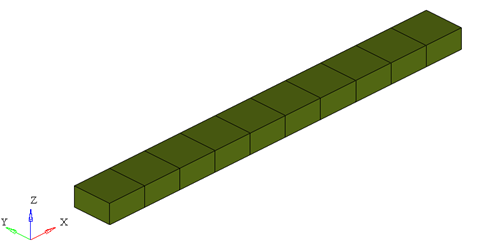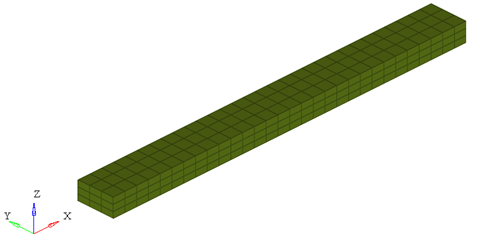# PBEAML

Geometric Properties ElementPBEAML lets you specify the geometric properties for an associated beam element of a specific cross section type.

## Format

<PBEAML
id       = "integer"
mid      = "integer"
type     = "string"
dim1a    = "real"
dim2a    = "real"
dim3a    = "real"
dim4a    = "real"
dim1b    = "real"
dim2b    = "real"
dim3b    = "real"
dim4b    = "real"
nx       = "integer"
ny       = "integer"
nz       = "integer"
ngx      = "integer"
ngy      = "integer"
ngz      = "integer"
graph    = "integer"
/>

## Attributes

id
Unique beam property identification number.
mid
Material property identification number.
dim1a, dim2a, dim3a, dim4a
Dimensions of the beam at the 1st node of the element.
type
Specifies the type of cross-section for this beam.
Choose from the following options:
• BAR, BOX, BOX1
• CHAN, CHAN1, CHAN2, CROSS
• H, HAT, I, I1, L, T, T1, T2 and Z

See 4 for more details on the cross-section types.

dim1b, dim2b, dim3b, dim4b
Dimensions of the beam at the second node of the element.
nx, ny, nz
Number of integration points in the X, Y and Z directions.
Default for nx is 5, ny is 3 and nz is 3.
ngx, ngy, ngz
Number of sub-elements in the X, Y and Z directions. The default for all three is 1. 5
graph
A post-processing flag that determines how this element is represented in the animation H3D. The default is 2. 5.

## Example

The example demonstrates the definition of a PBEAML property element.

<PBEAML id="1" mid="1" type="bar" dim1a="50.0" dim2a="50.0"   nx="5" ny="3" nz="3"/>

1. This type of property card is used to specify the geometric properties of the BEAM element. Each beam property element must have a unique identification number.
2. This property card defines the geometrical properties of the beam. The material properties of the beam are defined by the material specified by mid.
3. If only the dimensions at the start of the beam element are specified, MotionSolve assumes the cross section of the beam to be constant. However, if additionally, dimensions at the end of the beam element are specified, then these dimensions are varied linearly from node 1 to node 2 to represent the beam in the animation H3D.
4. The attribute type defines the type of cross section for this beam element. You may choose from the following types:
Type Cross section Required Inputs Default
BARdim1a,

dim2a

dim1b = dim1a,

dim2b = dim2a,

BOXdim1a,

dim2a,

dim3a,

dim4a

dim1b = dim1a,

dim2b = dim2a,

dim3b = dim3a,

dim4b = dim4a

BOX1dim1a, dim2a, dim3a,

dim4a

dim5a, dim6a

dim1b = dim1a,

dim2b = dim2a,

dim3b = dim3a,

dim4b = dim4a,

dim5b = dim5a,

dim6b = dim6a

CHANdim1a,

dim2a,

dim3a,

dim4a

dim1b = dim1a,

dim2b = dim2a,

dim3b = dim3a,

dim4b = dim4a

CHAN1dim1a,

dim2a,

dim3a,

dim4a
dim1b = dim1a,

dim2b = dim2a,

dim3b = dim3a,

dim4b = dim4a

CHAN2dim1a,

dim2a,

dim3a,

dim4a

dim1b = dim1a,

dim2b = dim2a,

dim3b = dim3a,

dim4b = dim4a

CROSSdim1a,

dim2a,

dim3a,

dim4a

dim1b = dim1a,

dim2b = dim2a,

dim3b = dim3a,

dim4b = dim4a

Hdim1a,

dim2a,

dim3a,

dim4a
dim1b = dim1a,

dim2b = dim2a,

dim3b = dim3a,

dim4b = dim4a

HATdim1a,

dim2a,

dim3a,

dim4a

dim1b = dim1a,

dim2b = dim2a,

dim3b = dim3a,

dim4b = dim4a

Idim1a,

dim2a,

dim3a,

dim4a

dim5a,

dim6a

dim1b = dim1a,

dim2b = dim2a,

dim3b = dim3a,

dim4b = dim4a,

dim5b = dim5a,

dim6b = dim6a

I1dim1a,

dim2a,

dim3a,

dim4a

dim1b = dim1a,

dim2b = dim2a,

dim3b = dim3a,

dim4b = dim4a
Ldim1a,

dim2a,

dim3a,

dim4a

dim1b = dim1a,

dim2b = dim2a,

dim3b = dim3a,

dim4b = dim4a

Tdim1a,

dim2a,

dim3a,

dim4a

dim1b = dim1a,

dim2b = dim2a,

dim3b = dim3a,

dim4b = dim4a

T1dim1a,

dim2a,

dim3a,

dim4a

dim1b = dim1a,

dim2b = dim2a,

dim3b = dim3a,

dim4b = dim4a

T2dim1a,

dim2a,

dim3a,

dim4a

dim1b = dim1a,

dim2b = dim2a,

dim3b = dim3a,

dim4b = dim4a

Zdim1a,

dim2a,

dim3a,

dim4a

dim1b = dim1a,

dim2b = dim2a,

dim3b = dim3a,

dim4b = dim4a
5. graph is a post-processing flag that determines how this element will be represented in the animation H3D file.
• graph = "0" implies that this element will not be represented in the H3D
• graph = "1" implies that this element will be represented as a line drawn between the two connecting nodes.
Note: When using graph="0" or graph="1", you will not be able to visualize the stress, strain or displacement contours. To do this, use graph="2" or graph="3".
• graph = "2" implies that the beam will be represented by 3D solid elements. This mode is useful when trying to visualize the stress/strain and displacement contours.
• graph = "3" implies that the beam is represented both as 3D solid elements as well as a line connecting the two nodes of the beam. This is useful when you need to visualize both the center line and the 3D representation of the beam.

When representing the beam as a solid, the arguments ngx, ngy and ngz determine the number of elements that are used to represent the beam in the animation H3D.ngx = ngy = ngz = 1ngx = ngy = ngz = 2ngx = ngy = ngz = 3

While increasing the ngx, ngy and ngz results in a better representation of the beam, it also increases the post-processing time taken by MotionSolve to write out the H3D. In addition, large values of ngx, ngy and ngz will increase the file size of the H3D considerably. Consider using the minimum values of these attributes that satisfy your visualization needs.